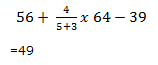# SSC CGL 2018 Practice Test Papers | Quantitative Aptitude (Day-26)

Dear Aspirants, Here we have given the Important SSC CGL Exam 2018 Practice Test Papers. Candidates those who are preparing for SSC CGL 2018 can practice these questions to get more confidence to Crack SSC CGL 2018 Examination.

[WpProQuiz 3086]

Click “Start Quiz” to attend these Questions and view Explanation

1) In a right angled isosceles triangle ABC, find the value of sinA + sinB + sinC.

a) 1

b) 1+√2

c) 1-√2

d) √2

2) The value of log tan 20o + log tan 45o + log tan 70o is ___

a) 1

b) 2

c) 3

d) 0

3) Find the angle of elevation of the sun, if the length of the shadow of a pole is 1/√3 times its height?

a) π/6

b) π/3

c) 2π/3

d) π/4

4) Find the area of a quadrant of a circle whose area is  equal to area of a square whose perimeter is 28 cm

a) 12.5

b) 13

c) 12.25

d) 14.5

5) A started  a business with Rs.3600 and B joins A with a investment of  Rs.  4800. When did B join, if the profit at the end of the year was divided in the ratio 3 : 2 ?

a) 6

b) 3

c) 9

d) 10

6) In a purse there are some notes of denominations Rs. 10, Rs. 20 and Rs.100. The number of Rs. 100  notes is equal to twice the number of notes of Rs. 20. The number of Rs. 10 notes is equal to thrice the number of  Rs. 100 notes. If the total amount in the purse is Rs. 840 find the number of Rs. 10 notes.

a) 20

b)18

c) 6

d) 16

7) The average age of a group of students is 16 years. If a 66 year old teacher joins the group, then the average increases by one year. What is the number of students in the group?

a) 50

b) 49

c) 30

d) 25

8) Wages of 30 women is Rs.60000 for 36 days. How many men must accompany 40 women to complete the job in 18 days within the budget Rs. 60000 and also a man earns double that of a woman.

a) 20

b) 18

c) 10

d) 25

9) Simplify: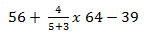a) 48

b) 49

c) 50

d) 55

10) Which of the following is true?

a) The sides of a regular polygon are not equal

b) A regular polygon can be inscribed in a circle.

c) The interior angle of a convex polygon is greater than 180o

d) All the above are true.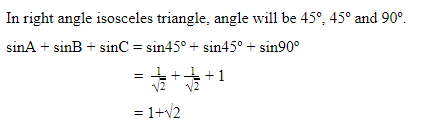log tan 20º + log tan 45º + log tan 70º

= log (tan 20º.tan 45º.tan 70º)

= log (tan 20º. cot 20º.1)

= log1 = 0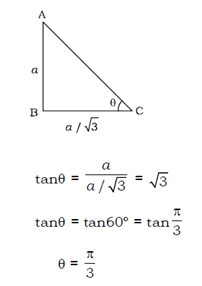Perimeter of square = 4a = 28

A  =7

Area of square = axa = 7×7 = 49

Area of Circle=  Area of square

Πr 2= a2

Πr2 = 49

Area of Quadrant = Πr2/4 = 49/4 = 12.25

Ratio of Profit = 3:2

Ratio of investment = 3:2

Investment of A = 3600*12

Investment of B = 4800*x

(3600*12)/(4800*x) = 3/2

X = 6

Let  the number of Rs. 10, Rs. 20 and Rs.100 be x, y and z respectively.

10x + 20y + 100z = 840——-(i)

Given: z = 2y——-(ii)

X= 3z => y = z/2——–(iii)

Sub (ii) and (iii) in (i)

30z+10z+100z=840

Z = 6

No. of Rs.10 notes, x=3 z=3×6 = 18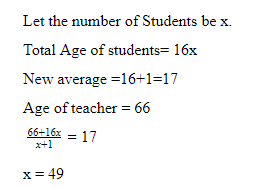Wages of 30 women for 36 days = 60000

Wages of 30 women for 18  day = 30000

Wage of 40 women for 18 day = 40000

Remaining amount = 60000 – 40000  = 20000

Wages of 20 women for 18 days = 20000

Since earning of man is twice that of a women ,10 men must accompany 40 Women to complete the job in 18 days.